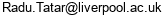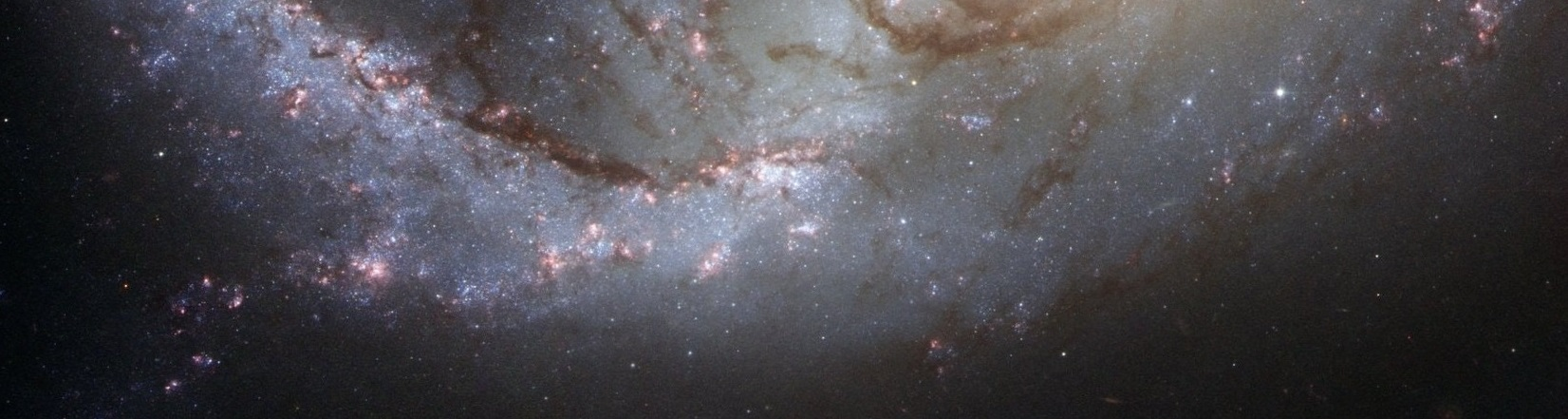"The universe cannot be read until we have learnt the language and become familiar with the characters in which it is written. It is written in mathematical language, and the letters are triangles, circles and other geometrical figures, without which means it is humanly impossible to comprehend a single word".

Galileo Galilei - Il Saggiatore (1623)

MEMBERS

DFT (IFIN-HH)

• Elena Mirela Babalic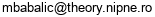String theory, mathematical physics
• Stefan Berceanu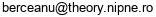Geometric quantization, geometric representation theory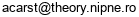Integrable systems, algebraic geometry
• Calin Iuliu Lazaroiu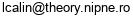String theory, symplectic and algebraic geometry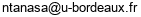Combinatorial physics, random models
• Mihai Visinescu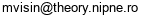Quantum field theory, gravitation, field theory on curved spaces, mathematical physics

IMAR

• Florin Ambro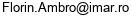Algebraic Geometry
• Marian Aprodu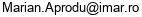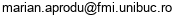Algebraic Geometry
• Daniel Beltita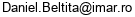Operator algebras, representation theory
• Vasile Brinzanescu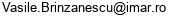Algebraic geometry
• Liana David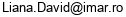Differential geometry
• Cezar Joita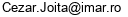Complex analysis and singularity theory
• Liviu Ornea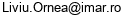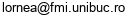Differential geometry
• Sergiu Moroianu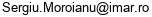Differential geometry

Other Romanian institutions

• Corina Nicoleta Babalic (Univ. of Craiova)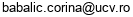Integrable systems
• Eugen Mihaita Cioroianu (Univ. of Craiova)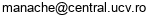BRST cohomologies, QFT, differential geometry
• Cornelia Vizman (West University, Timisoara)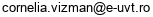symplectic geometry, diffeomorphism groups
• Victor Vuletescu (Univ. of Bucharest)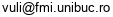Algebraic geometry

International

• Lilia Anguelova (INRNE, Sofia)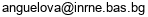String Theory
• Iosif Razvan Bena (IPhT-CEA, Saclay)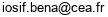String theory, gauge/gravity duality
• Andrei Caldararu (Univ. Wisconsin)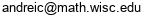Derived algebraic geometry, string theory
• Ionut Ciocan-Fontanine (Univ. of Minnesota)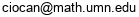Algebraic geometry, moduli spaces, Gromov-Witten theory
• Ioana Alexandra Coman-Lohi  (Univ. Amsterdam)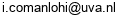Topological string theory, conformal field theory
• Emilian Dudas (E. Polytechnique, Paris)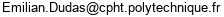String phenomenology
• Razvan Gurau (CNRS, CPHT & Perimeter Institute)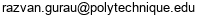Tensor models, nonperturbative QFT
• Leonardo Mihalcea (Virginia Tech. Univ.)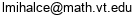Algebraic geometry, integrable systems, representation theory
• Andrei Moroianu (U. Paris-Sud)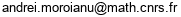Differential geometry
• Andrei Negut (MIT)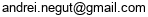Algebraic geometry, representation theory, quantum algebras
• Tudor Ratiu (Shanghai Jiao Tong Univ.)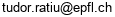Geometric analysis
• Radu Roiban (Penn. State Univ.)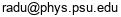String theory, supergravity
• Didina Serban (IPhT-CEA, Saclay)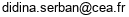Integrability, gauge-gravity duality